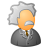# QlikView App Dev

Discussion Board for collaboration related to QlikView App Development.

cancel
Showing results for
Did you mean:Creator III

## How to write script for the given condition?

Hello,

I have created a backend script for my filter as;

If(Code = '3', 'Mechanical',

If(Code = '4', 'Controls',

If(Code = '5', 'System'))) as Engineering,

But I want to create another condition combining Code = '3', Code = '4', Code = '5' as Total in the same Engineering Category.

Any idea on how to do this?

thanks

Bhavesh

1 Solution

Accepted SolutionsMVP

Concatenate to the above table

from ...

where Match(Code, 3, 4, 5);

2 RepliesMVP

Concatenate to the above table

from ...

where Match(Code, 3, 4, 5);Creator III
Author

thank you stalwar1‌,  I have now Total added as another condition for my filter "Engineering". But the Expression for my Sales is not filtering. This is how my expression is;

=if(Code = '3', Sum(Sales_3),

if(Code = '4', Sum(Sales_4),

if(Code = '5', Sum(Sales_5))))

//Script:

y:

*,

If(Code = '3', 'Mechanical',

If(Code = '4', 'Controls',

If(Code = '5', 'System'))) as Engineering,

Resident x;

Left Join (y)

if(Cost_Code = '3', Sales,0) as Sales_3,

if(Cost_Code = '4', Sales,0) as Sales_4,

if(Cost_Code = '5', Sales,0) as Sales_5,

Resident y